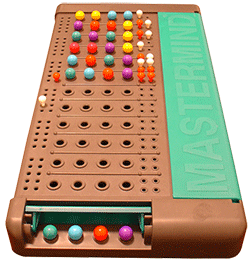# Informed Mastermind guessing with Max-PartsRecently I came across a programming challenge which required me to write a mastermind solver. Basically there would be a server which hosts the game and replies to the user's guesses. Of course I wanted to automate the whole guessing process and solve Mastermind algorithmically.

## Problem Description

Mastermind is a color guessing game in which one person has a secret combination of colors and the other one has to make guesses to eventually be able to infer the secret. The secret-holder replies to a guess with which pieces are at the right place with the right color and how many of the other pieces' colors are part of the secret but at the wrong place. The reply is usually represented with red and white pins. The difficulty of the game depends on the number of colors available and the number of colors the secret consists off. There are actually a lot of different Mastermind games but the challenge focused on Super-Mastermind with eight colors in five spots yielding a total of $8^5 = 32768$ possible solutions. The maximum number of guesses was 35.

## Algorithm

From here on out I will be referring to the possible colors with the integer values $[0, 7]$.

The first guess is actually quite important for the algorithm. Because of this I ran a simple script which evaluated all possible starting values and used the one which gave the best results. I basically checked all possible initial values against $2000$ randomly generated games hosted by the server. Obviously the games were only generated once and not for each initial value. It turned out that the optimal starting value was ${0, 0, 1, 2, 3}$.

After the first guess the obvious next step would be to discard every possible solution that doesn't match the result. But how do we do that?

Given that we know our previous query and the result ( = the number red/white pins) we can discard possible solutions which, given that they were the solution, would not yield the same result for our previous input.

Now we need to select a new solution. My first step was to divide the remaining possibilities into partitions. The partition of a solution is based on the number of different red/white pin-combinations that it would generate if it were the answer and all the other remaining solutions were the guess. I then select one solution which is part of the partition with the highest number. This also explains name of the optimization - Max-Part.

### Performance Considerations

One part of the challenge was that the time limit in which the game had to end was two seconds. Due to this limitation I couldn't use the Max-Parts optimization from the beginning because of the high time complexity of $O(n^2)$, where n is the number of remaining solutions. After the first guess I usually had $~10000$ possibilities left, which is why I only used Max-Parts after the number of solutions left had been reduced drastically to $~2000$.

## Statistics

Using this algorithm I achieved a mean of $5.683$ which was quite satisfactory and actually below the mean for an implementation of Knuth's algorithm (the maximum number of guesses is worse though).

 Mean $5.683$ Median $6.000$ Stddev $0.865$
Guesses Occurrences
$1$ $1$
$2$ $19$
$3$ $238$
$4$ $2165$
$5$ $10504$
$6$ $15033$
$7$ $4387$
$8$ $401$
$9$ $20$

## The Code

I wrote the solver under Linux with C. The most interesting parts of the source-code follow.

#### Enumerate all possible solutions

static void enumeratePossibilities(uint8_t level) {
int i;
for(i = 0; i < COLORS; i++) {
choice[level] = i;
if(level != (SLOTS - 1)) {
enumeratePossibilities(level + 1);
} else {
int j;
for(j = 0; j < SLOTS; j++) {
allPossibilities[enumIndex][j] = choice[j];
}
enumIndex++;
}
}
}


#### Calculate the result given a secret and a guess

static void getResults(uint8_t *guess, uint8_t *secret, uint8_t *result) {
int colors_left[COLORS];
int red, white, j;

/* marking red and white */
(void) memset(&colors_left, 0, sizeof(colors_left));
red = white = 0;
for (j = 0; j < SLOTS; ++j) {
/* mark red */
if (guess[j] == secret[j]) {
red++;
} else {
colors_left[secret[j]]++;
}
}
for (j = 0; j < SLOTS; ++j) {
/* not marked red */
if (guess[j] != secret[j]) {
if (colors_left[guess[j]] > 0) {
white++;
colors_left[guess[j]]--;
}
}
}

result = red;
result = white;
}


#### Discard invalid solutions

for(i = 0; i < SOLUTION_CNT; i++) {
if(possibleSolutionNumbers[i] == 0) {
getResults(allPossibilities[i], guess, result);
if(result != red || result != white) {
possibleSolutionNumbers[i] = -1;
discaredSolutions++;
}
}
}


#### Advanced selections using Max-Parts

/* only use advanced selection if the number of discarded solution
is high enough this would require much more than 1 second if
it was performed with 32768 solutions */
if(discaredSolutions > 30500) {
int maxPart = 0, part;
for(i = 0; i < SOLUTION_CNT; i++) {
if(possibleSolutionNumbers[i] == 0) {
part = getPartitions(allPossibilities[i]);
if(part > maxPart) {
nextGuess = i;
maxPart = part;
}
}
}
}


#### Returns the number of partitions of one guess

static int getPartitions(uint8_t *guess) {
uint8_t result;
int partitions[SLOTS][SLOTS] = { { 0 } };
int i = 0, ret = 0;

for(i = 0; i < SOLUTION_CNT; i++) {
if(possibleSolutionNumbers[i] == 0) {
getResults(guess, allPossibilities[i], result);
if(partitions[result][result] == 0) {
partitions[result][result] = -1;
ret++;
}
}
}
return ret;
}